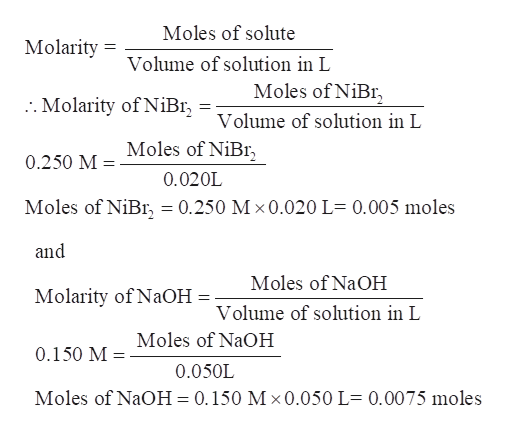# how do i find the total mass of percipitated product produced in a reaction where i have 50 ml sample of .150 M NaOH is added to 20.0 mL of .250 M NiBr2? step by step please

Question

how do i find the total mass of percipitated product produced in a reaction where i have 50 ml sample of .150 M NaOH is added to 20.0 mL of .250 M NiBr2? step by step please

check_circleExpert Solution
Step 1

We have to calculate the mass of the product that forms when 50 ml sample of 0.150 M NaOH is added to 20.0 mL of 0.250 M NiBr2.

Step 2

Nickel bromide reacts with NaOH to form the precipitate of nickel hydroxide.

Step 3

Calculate the moles of reactants, NiBr2 and NaOH, using their molarity and volume.

Molarity of NiBr2= 0.250 M

Volume of NiBr2 solution= ...help_outlineImage TranscriptioncloseMoles of solute Molarity Volume of solution in L Moles of NiBr :. Molarity of NiBI Volume of solution in L Moles of NiBr 0.250 M 0.020L Moles of NiBr, = 0.250 M x0.020 L- 0.005 moles and Moles of NaOH Molarity of NaOH Volume of solution in L Moles of NaOH 0.150 M 0.050L Moles of NaOH 0.150 M x 0.050 L 0.0075 moles fullscreen

### Want to see the full answer?

See Solution

#### Want to see this answer and more?

Solutions are written by subject experts who are available 24/7. Questions are typically answered within 1 hour*

See Solution
*Response times may vary by subject and question
Tagged in

### General Chemistry